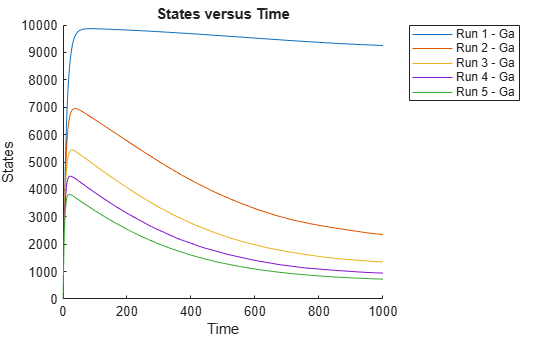# Perform a Parameter Scan

This example shows how to perform a parameter scan by simulating a model multiple times, each time varying the value of a parameter.

In the model described in Model of the Yeast Heterotrimeric G Protein Cycle, the rate of G protein inactivation (`kGd`) is much lower in the mutant strain versus the wild-type strain (`kGd = 0.004` versus `kGd = 0.11`), which explains higher levels of activated G protein (`Ga`) in the mutant strain. For a detailed look at how varying the level of `kGd` affects the level of `Ga`, perform a parameter scan over different values of `kGd`.

Load the `gprotein.sbproj` project, which includes the variable `m1`, a model object.

`sbioloadproject gprotein`

Create a vector of five evenly spaced values for `kGd` ranging from `0.001` to `0.15`.

`kGdValues = linspace(1e-3,0.15,5)';`

Create a `SimFunction` object, where `kGd` is the input parameter to scan, and `Ga` is the observed species. Pass in an empty array [] as the last input argument to denote there are no dosed species.

`simfunc = createSimFunction(m1,{'kGd'},{'Ga'},[]);`

Simulate the model multiple times with different kGd values. Set the stop time to 1000.

`sd = simfunc(kGdValues,1000);`

Plot the simulation results to see how varying the level of `kGd` affects the level of `Ga`.

`sbioplot(sd);`